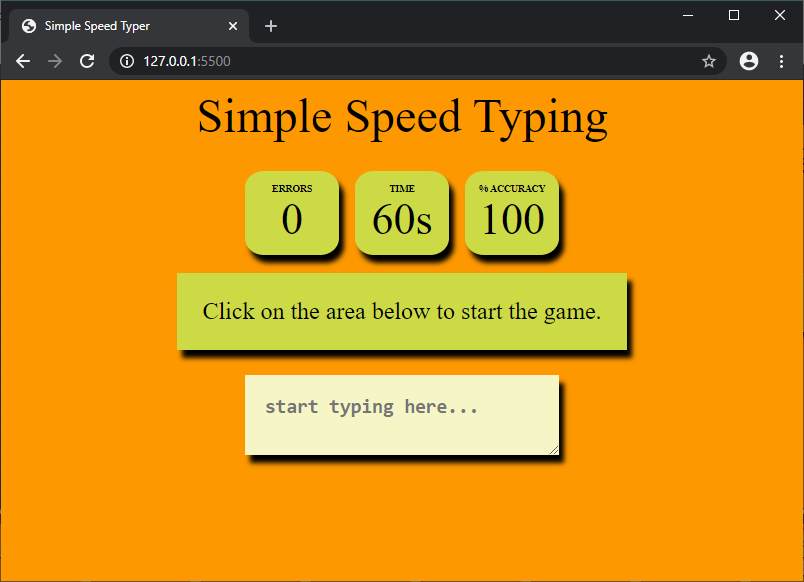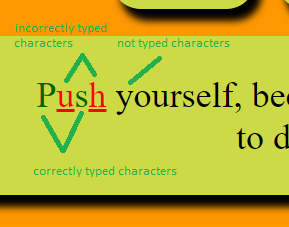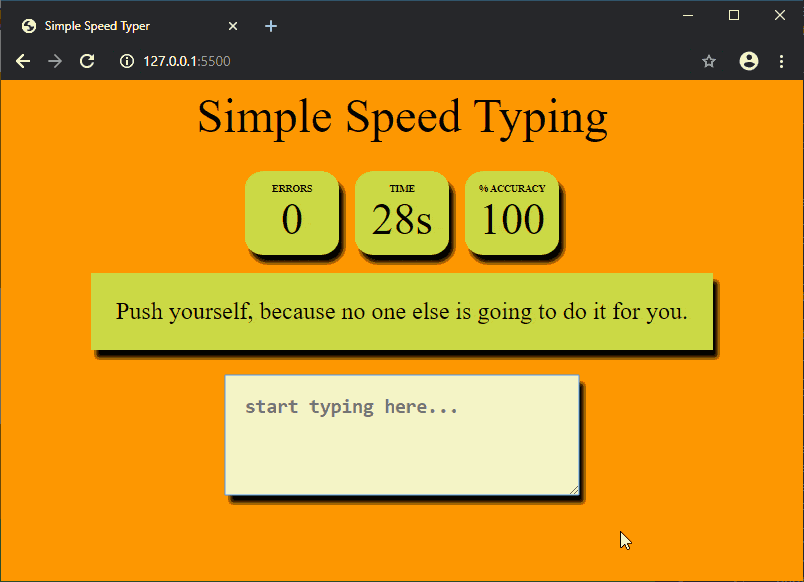# Design a typing speed test game using JavaScript

A typing test is designed to find how fast one types in a given amount of time. We will be designing a typing game using JavaScript that presents a simple typing challenge and finds the performance of typing by calculating the Characters Per Minute (CPM), Words Per Minute (WPM) and the accuracy of the typed characters.

The game shows a series of quotes that have to be typed in a specified time limit, as fast as possible. A higher typing speed would show a higher WPM value. Incorrectly typed characters would be marked accordingly during typing.

We will create the HTML layout first, style it using CSS and then write the logic in JavaScript.
The HTML Layout: The HTML layout defines the element structure that would be shown on the page. This includes:

• Header Portion: This section shows the statistics of the current typing session. This includes the display of the time left, number of errors, accuracy, WPM and CPM.
• Quote Section: This section shows the current text that has to be typed in the input area.
• Input Area: This section contains the input area where the text has to be typed.
• Restart Button: This is the restart button which would be shown once the time runs out and the game finishes.
• Code:

 `<``html` `lang``=``"en"``> ` `<``head``> ` `    ``<``title``>Simple Speed Typer ` ` `  `    ``` `    ``<``link` `rel``=``"stylesheet"` `href``=``"style.css"``> ` ` ` `<``body``> ` `  ``<``div` `class``=``"container"``> ` `    ``<``div` `class``=``"heading"``> ` `      ``Simple Speed Typing ` `    `` ` `    ``<``div` `class``=``"header"``> ` `      ``<``div` `class``=``"wpm"``> ` `        ``<``div` `class``=``"header_text"``>WPM ` `        ``<``div` `class``=``"curr_wpm"``>100 ` `      `` ` `      ``<``div` `class``=``"cpm"``> ` `        ``<``div` `class``=``"header_text"``>CPM ` `        ``<``div` `class``=``"curr_cpm"``>100 ` `      `` ` `      ``<``div` `class``=``"errors"``> ` `        ``<``div` `class``=``"header_text"``>Errors ` `        ``<``div` `class``=``"curr_errors"``>0 ` `      `` ` `      ``<``div` `class``=``"timer"``> ` `        ``<``div` `class``=``"header_text"``>Time ` `        ``<``div` `class``=``"curr_time"``>60s ` `      `` ` `      ``<``div` `class``=``"accuracy"``> ` `        ``<``div` `class``=``"header_text"``>% Accuracy ` `        ``<``div` `class``=``"curr_accuracy"``>100 ` `      `` ` `    `` ` ` `  `    ``<``div` `class``=``"quote"``> ` `      ``Click on the area below to start the game. ` `    `` ` `    ``<``textarea` `class``=``"input_area"` `      ``placeholder``=``"start typing here..."` `      ``oninput``=``"processCurrentText()"` `      ``onfocus``=``"startGame()"``> ` `    `` ` `    ``<``button` `class``=``"restart_btn"` `      ``onclick``=``"resetValues()"``> ` `      ``Restart ` `    `` ` `  `` ` ` `  `  ``` `  ``<``script` `src``=``"game.js"``> ` `  `` ` ` ` ` `

Note: Each of the portions is filled with dummy data to make styling easier. The HTML code of the above is as follows.

The CSS Styling: CSS is used to style the different portions and make it more visually appealing.

• The header portion is displayed using the flex layout.
• The text size of each element is such that it is easily readable by the user when playing the game.
• Two additional classes are defined to denote the letters that are typed correctly or incorrectly. These classes would be dynamically added or removed when required.
• Code:

 `body { ` `  ``background-color: #fe9801; ` `  ``color: black; ` `  ``text-align: center; ` `} ` ` `  `.container { ` `  ``display: flex; ` `  ``flex-direction: column; ` `  ``align-items: center; ` `} ` ` `  `.heading { ` `  ``margin-bottom: 20px; ` `  ``font-size: 3rem; ` `  ``color: black; ` `} ` ` `  `.header { ` `  ``display: flex; ` `  ``align-items: center; ` `} ` ` `  `.timer, .errors, .accuracy, ` `.cpm, .wpm { ` `  ``background-color: #ccda46; ` `  ``height: 60px; ` `  ``width: 70px; ` `  ``margin: 8px; ` `  ``padding: 12px; ` `  ``border-radius: 20%; ` `  ``box-shadow: black 5px 8px 5px; ` `} ` ` `  `.cpm, .wpm  { ` `  ``display: none; ` `} ` ` `  `.header_text { ` `  ``text-transform: uppercase; ` `  ``font-size: 0.6rem; ` `  ``font-weight: 600; ` `} ` ` `  `.curr_time, .curr_errors, ` `.curr_accuracy, .curr_cpm, ` `.curr_wpm { ` `  ``font-size: 2.75rem; ` `} ` ` `  `.quote { ` `  ``background-color: #ccda46; ` `  ``font-size: 1.5rem; ` `  ``margin: 10px; ` `  ``padding: 25px; ` `  ``box-shadow: black 5px 8px 5px; ` `} ` ` `  `.input_area { ` `  ``background-color: #f5f5c6; ` `  ``height: 80px; ` `  ``width: 40%; ` `  ``font-size: 1.5rem; ` `  ``font-weight: 600; ` `  ``margin: 15px; ` `  ``padding: 20px; ` `  ``border: 0px; ` `  ``box-shadow: black 5px 8px 5px; ` `} ` ` `  `.restart_btn { ` `  ``display: none; ` `  ``background-color: #326765; ` `  ``font-size: 1.5rem; ` `  ``padding: 10px; ` `  ``border: 0px; ` `  ``box-shadow: black 5px 8px 5px; ` `} ` ` `  `.incorrect_char { ` `  ``color: red; ` `  ``text-decoration: underline; ` `} ` ` `  `.correct_char { ` `  ``color: darkgreen; ` `} `

The result of the HTML layout and CSS styling would look like this:Main Logic of the game: The main logic of the game is defined in a JavaScript file. There are several functions that work together to run the game.

Step 1: Selecting all the elements and defining variables

The required elements in the HTML layout are first selected using the `querySelector()` method. They are assigned variable names so that they could be easily accessed and modified. Other variables that would be accessed throughout the program are also defined in the beginning.

 `// define the time limit ` `let TIME_LIMIT = 60; ` ` `  `// define quotes to be used ` `let quotes_array = [ ` `  ``"Push yourself, because no one else is going to do it for you."``, ` `  ``"Failure is the condiment that gives success its flavor."``, ` `  ``"Wake up with determination. Go to bed with satisfaction."``, ` `  ``"It's going to be hard, but hard does not mean impossible."``, ` `  ``"Learning never exhausts the mind."``, ` `  ``"The only way to do great work is to love what you do."` `]; ` ` `  `// selecting required elements ` `let timer_text = document.querySelector(``".curr_time"``); ` `let accuracy_text = document.querySelector(``".curr_accuracy"``); ` `let error_text = document.querySelector(``".curr_errors"``); ` `let cpm_text = document.querySelector(``".curr_cpm"``); ` `let wpm_text = document.querySelector(``".curr_wpm"``); ` `let quote_text = document.querySelector(``".quote"``); ` `let input_area = document.querySelector(``".input_area"``); ` `let restart_btn = document.querySelector(``".restart_btn"``); ` `let cpm_group = document.querySelector(``".cpm"``); ` `let wpm_group = document.querySelector(``".wpm"``); ` `let error_group = document.querySelector(``".errors"``); ` `let accuracy_group = document.querySelector(``".accuracy"``); ` ` `  `let timeLeft = TIME_LIMIT; ` `let timeElapsed = 0; ` `let total_errors = 0; ` `let errors = 0; ` `let accuracy = 0; ` `let characterTyped = 0; ` `let current_quote = ``""``; ` `let quoteNo = 0; ` `let timer = ``null``; `

Step 2: Preparing the text to be displayed

A function `updateQuote()` is defined which handles the following things:

• Getting the text
Quotes have been used as the text that has to be typed to play the game. Each quote is taken one by one from a predefined array. A variable keeps track of the current quote index and increments it whenever a new one is requested.
• Splitting the characters into elements
Each of the characters in the text is separated into a series of `<span>` elements. This makes it possible to individually change the color of each character depending upon if it has been correctly typed by the user. These elements are appended to a variable `quote_text`.

 `function` `updateQuote() { ` `  ``quote_text.textContent = ``null``; ` `  ``current_quote = quotes_array[quoteNo]; ` ` `  `  ``// separate each character and make an element  ` `  ``// out of each of them to individually style them ` `  ``current_quote.split(``''``).forEach(char => { ` `    ``const charSpan = document.createElement(``'span'``) ` `    ``charSpan.innerText = char ` `    ``quote_text.appendChild(charSpan) ` `  ``}) ` ` `  `  ``// roll over to the first quote ` `  ``if` `(quoteNo < quotes_array.length - 1) ` `    ``quoteNo++; ` `  ``else` `    ``quoteNo = 0; ` `} `

Step 3: Getting the currently typed text by the user

A function `processCurrentText()` is defined which will be invoked whenever the user types or changes anything in the input box. It is hence used with the `oninput` event handler of the input box. This function handles the following things:

• Getting the current value of the input box
The `value` property of the input area is used to get the current text typed by the user. This is split into an array of characters to compare with the quote text. This is stored in `curr_input_array`.
• Coloring the characters of the quote text
The characters of the displayed quote are colored ‘red’ or ‘green’ depending on whether it has been typed correctly. This is done by selecting the span elements of the quote we have created earlier and looping through them. The element has then applied the classes created above depending on if it matches the typed text.
• Calculating the errors and accuracy
Every time the user makes a mistake during typing, the `errors` variable is incremented. This is used to calculate the accuracy value by dividing the number of correctly typed characters with the total number of characters typed by the user.
• Moving to next quote
When the length of the input text matches the quote text length, the `updateQuote()` function is called which changes the quote and clears the input area. The number of total errors is also updated to be used for the next quote.

 `function` `processCurrentText() { ` ` `  `  ``// get current input text and split it ` `  ``curr_input = input_area.value; ` `  ``curr_input_array = curr_input.split(``''``); ` ` `  `  ``// increment total characters typed ` `  ``characterTyped++; ` ` `  `  ``errors = 0; ` ` `  `  ``quoteSpanArray = quote_text.querySelectorAll(``'span'``); ` `  ``quoteSpanArray.forEach((char, index) => { ` `    ``let typedChar = curr_input_array[index] ` ` `  `    ``// character not currently typed ` `    ``if` `(typedChar == ``null``) { ` `      ``char.classList.remove(``'correct_char'``); ` `      ``char.classList.remove(``'incorrect_char'``); ` ` `  `      ``// correct character ` `    ``} ``else` `if` `(typedChar === char.innerText) { ` `      ``char.classList.add(``'correct_char'``); ` `      ``char.classList.remove(``'incorrect_char'``); ` ` `  `      ``// incorrect character ` `    ``} ``else` `{ ` `      ``char.classList.add(``'incorrect_char'``); ` `      ``char.classList.remove(``'correct_char'``); ` ` `  `      ``// increment number of errors ` `      ``errors++; ` `    ``} ` `  ``}); ` ` `  `  ``// display the number of errors ` `  ``error_text.textContent = total_errors + errors; ` ` `  `  ``// update accuracy text ` `  ``let correctCharacters = (characterTyped - (total_errors + errors)); ` `  ``let accuracyVal = ((correctCharacters / characterTyped) * 100); ` `  ``accuracy_text.textContent = Math.round(accuracyVal); ` ` `  `  ``// if current text is completely typed ` `  ``// irrespective of errors ` `  ``if` `(curr_input.length == current_quote.length) { ` `    ``updateQuote(); ` ` `  `    ``// update total errors ` `    ``total_errors += errors; ` ` `  `    ``// clear the input area ` `    ``input_area.value = ``""``; ` `  ``} ` `} `Coloring of the characters based its correctness

Step 4: Starting a new game

A function `startGame()` is defined which will be invoked when the user focuses on the input box. It is hence used with the `onfocus` event handler of the input box. This function handles the following things:

• Reset all values
All the values are reset to their default ones before the starting of a new game. We create a different function named `resetValues()` which handles this.
• Update the quote text
A new quote text is made ready and displayed by calling the `updateQuote()` function.
• Creating a new timer
A timer keeps track of the number of seconds left and displays it to the user. It is created using the `setInterval()` method which repeatedly calls the `updateTimer()` function defined below. Before creating a new timer, the previous timer instance is cleared using `clearInterval()`.

 `function` `startGame() { ` ` `  `  ``resetValues(); ` `  ``updateQuote(); ` ` `  `  ``// clear old and start a new timer ` `  ``clearInterval(timer); ` `  ``timer = setInterval(updateTimer, 1000); ` `} ` ` `  `function` `resetValues() { ` `  ``timeLeft = TIME_LIMIT; ` `  ``timeElapsed = 0; ` `  ``errors = 0; ` `  ``total_errors = 0; ` `  ``accuracy = 0; ` `  ``characterTyped = 0; ` `  ``quoteNo = 0; ` `  ``input_area.disabled = ``false``; ` ` `  `  ``input_area.value = ``""``; ` `  ``quote_text.textContent = ``'Click on the area below to start the game.'``; ` `  ``accuracy_text.textContent = 100; ` `  ``timer_text.textContent = timeLeft + ``'s'``; ` `  ``error_text.textContent = 0; ` `  ``restart_btn.style.display = ``"none"``; ` `  ``cpm_group.style.display = ``"none"``; ` `  ``wpm_group.style.display = ``"none"``; ` `} `

Step 5: Updating the timer

A function `updateTimer()` is defined which will be invoked every second to keep track fo the time. This function handles the following things:

• Update the time values
All the variables that keep track of the time are updated. The `timeLeft` value is decremented, the `timeElapsed` value is incremented, and the timer text is updated to the current time left.
• Finishing the game
This portion is triggered when the time limit is reached. It calls the `finishGame()` function defined below which finishes the game.

 `function` `updateTimer() { ` `  ``if` `(timeLeft > 0) { ` `    ``// decrease the current time left ` `    ``timeLeft--; ` ` `  `    ``// increase the time elapsed ` `    ``timeElapsed++; ` ` `  `    ``// update the timer text ` `    ``timer_text.textContent = timeLeft + ``"s"``; ` `  ``} ` `  ``else` `{ ` `    ``// finish the game ` `    ``finishGame(); ` `  ``} ` `} `

Step 6: Finshing the game

A function `finishGame()` is defined which will be invoked when the game has to be finished. This function handles the following things:

• Deleting the timer
The timer instance created before is removed.
• Displaying the restart game text and button
The quoted text displayed to the user is changed to one that indicates that the game is over. The ‘Restart’ button is also displayed by setting the display property to ‘block’.
• Calculating the CPM and WPM of the current session
1. The Characters Per Minute (CPM) is calculated by dividing the total number of characters typed with the time elapsed and then multiplying the result with 60. It is rounded off to prevent decimal points.
2. The Words Per Minute (WPM) is calculated by dividing the CPM by 5 and then multiplying the result with 60. The 5 denotes the average number of characters per word. It is rounded off to prevent decimal points.

 `function` `finishGame() { ` `  ``// stop the timer ` `  ``clearInterval(timer); ` ` `  `  ``// disable the input area ` `  ``input_area.disabled = ``true``; ` ` `  `  ``// show finishing text ` `  ``quote_text.textContent = ``"Click on restart to start a new game."``; ` ` `  `  ``// display restart button ` `  ``restart_btn.style.display = ``"block"``; ` ` `  `  ``// calculate cpm and wpm ` `  ``cpm = Math.round(((characterTyped / timeElapsed) * 60)); ` `  ``wpm = Math.round((((characterTyped / 5) / timeElapsed) * 60)); ` ` `  `  ``// update cpm and wpm text ` `  ``cpm_text.textContent = cpm; ` `  ``wpm_text.textContent = wpm; ` ` `  `  ``// display the cpm and wpm ` `  ``cpm_group.style.display = ``"block"``; ` `  ``wpm_group.style.display = ``"block"``; ` `} `

Final Demonstration

The game is now ready to be played in any browser.My Personal Notes arrow_drop_upCheck out this Author's contributed articles.

If you like GeeksforGeeks and would like to contribute, you can also write an article using contribute.geeksforgeeks.org or mail your article to contribute@geeksforgeeks.org. See your article appearing on the GeeksforGeeks main page and help other Geeks.

Please Improve this article if you find anything incorrect by clicking on the "Improve Article" button below.